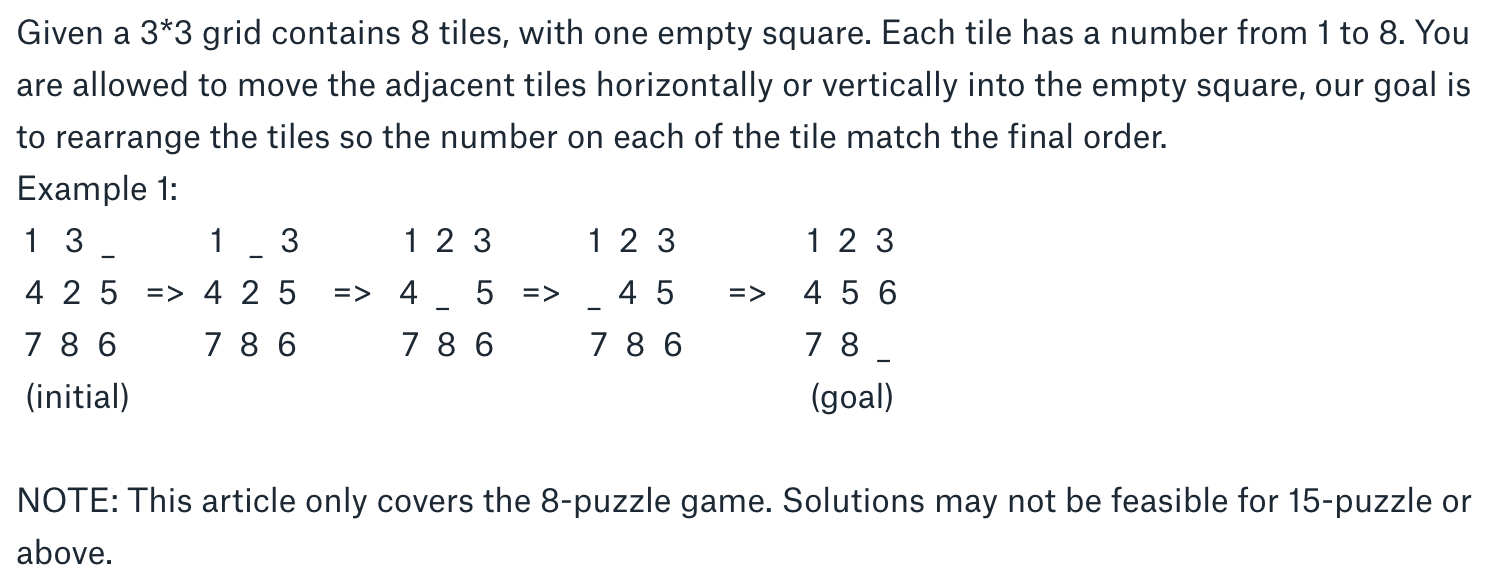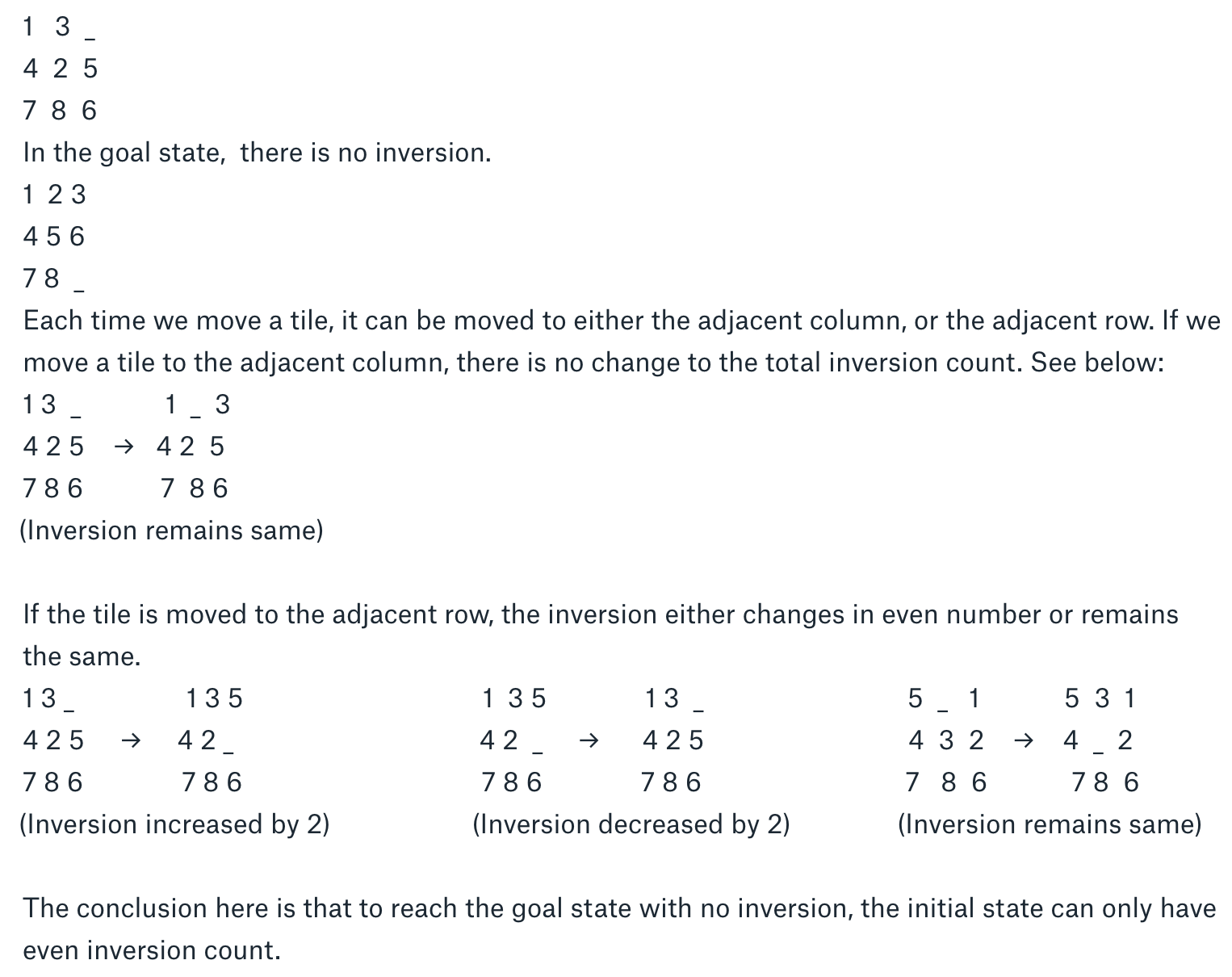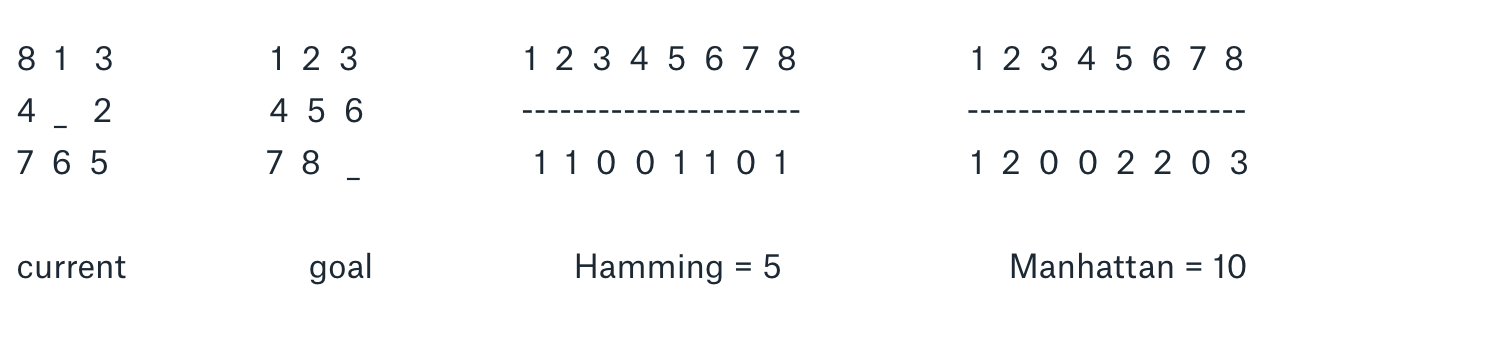# The 8-Puzzle Game (Airbnb Hard)

Given a 3*3 grid contains 8 tiles, with one empty square. Each tile has a number from 1 to 8. You are allowed to move the adjacent tiles horizontally or vertically into the empty square, our goal is to rearrange the tiles so the number on each of the tile match the final order.Is the puzzle solvable?

Given a random initial state, it is not always possible to reach the goal state by a series of slides. A mathematics way to detect an infeasible puzzle state is counting the inversions of the numbers. If two values on the tiles are in reverse order, it forms a pair of inversion. for example in Example 1, by comparing the numbers one by one, we count total up to 4 pairs of inversions in the initial state. (3,2) (4,2)(7,6)(8,6)``````
public boolean isSolvable(int[][] initialState) {
int inversionCount = 0;
int[] arr = flatten2D(initialState);
for(int i=0; i < 8; i++) {
for(int j = i+1; j< 9; j++) {
if(arr[j] != 0 && arr[i] != 0 && arr[i] > arr[j])
inversionCount++;
}
}
return inversionCount % 2 ==0;
}
public int[] flatten2D(int[][] input) {
int[] res = new int;
int k = 0;
for(int i=0; i < 3; i++) {
for(int j = 0; j < 3; j++) {
res[k++] = input[i][j];
}
}
return res;
}
``````

Pathfinding with the A* algorithm

Given a solvable initial state, there are several ways can be implemented to find the path towards the goal state. A* algorithm is a popular algorithm used widely in pathfinding, it is a best-first search based recursive algorithm. For each state in between initial state and goal state, to select the best move from all possible movements, A* algorithm introduces a heuristic formula that helps make the decision.
f(n) = g(n)+h(n)
where n is the current sate on the path we are examining, g(n) is the cost of the path from the initial state to n, and h(n) is a heuristic that estimates the cost of the cheapest path from n to the goal state.

In our case, g(n) is the numbers of steps has been moved so far from initial state to current state. There are several priority functions can be used to calculate the h(n):

• Hamming Distance: The number of tiles not in their goal position.
• Manhattan Distance: The sum of the vertical and horizontal distances from each tile to their goal position.``````
import java.util.*;
public class EightPuzzleAStar {
public static final int[][] DIR  = {{0,1},{1,0},{0,-1},{-1,0}};
public int len;

public void solvePuzzle(int[][] initialState,int[][] finalState) {
int x =0, y = 0;
for(int i=0; i < len; i++) {
for(int j=0; j < len; j++) {
if(initialState[i][j] == 0) {
x = i;
y = j;
}
}
}  //find the position of empty space

PriorityQueue< State > pq = new PriorityQueue<>();
State root = new State(initialState, x,y, x,y, 0, null);
root.cost = calculateHammingCost(initialState, finalState);

pq.offer(root);
while(!pq.isEmpty()) {
State current = pq.poll();
if(current.cost == 0) {
printPath(current);
System.out.println("Total steps: "+current.step);
return;
}//reach the goal state
for(int[] dir : DIR) {
int newX = current.x+dir;
int newY = current.y+dir;

if(isSafe(newX, newY)) {
State child = new State(current.matrix, current.x,current.y, newX, newY, current.step+1, current);
if(current.parent == null || !getString(current.parent.matrix).equals(getString(child.matrix))) {
child.cost = calculateHammingCost(child.matrix, finalState);
pq.offer(child);
}
}
}
}
}
public boolean isSafe(int x, int y){
return (x >= 0 && x < len && y >= 0 && y < len);
}
public int calculateHammingCost(int[][] currentState, int[][] finalState) {
int cost = 0;
for(int i=0; i < len;i++) {
for(int j = 0; j < len;j++) {
if(currentState[i][j]!= 0 && currentState[i][j] != finalState[i][j]) {
cost++;
}
}
}
return cost;
}//calculate the cost using Hamming distance

public int calculateManhattanCost(int[][] currentState, int[][] finalState) {
int cost = 0;
String current = getString(currentState);
String goal = getString(finalState);
for(int i=1; i< 9; i++) {
int indexCurrent = current.indexOf(String.valueOf(i));
int indexGoal = goal.indexOf(String.valueOf(i));
cost += Math.abs(indexCurrent / len - indexGoal / len)
+ Math.abs(indexCurrent % len - indexGoal % len);
}
return cost;
}//calculate the cost using Manhattan distance

public String getString(int[][] matrix) {
String result = "";
for(int i=0; i < len; i++) {
for(int j=0; j < len ; j++) {
result += matrix[i][j]+",";
}
}
return result;
}
public void printPath(State root) {
if(root == null) {
return;
}
printPath(root.parent);
printMax(root.matrix);
System.out.println();
}
public void printMax(int[][] matrix) {
for(int i=0; i < len;i++) {
for(int j= 0; j < len; j++) {
System.out.print(matrix[i][j]);
}
System.out.println();
}
}
public static void main(String[] args) {
EightPuzzleAStar eightPuzzle = new EightPuzzleAStar();
int[][] initialState = { {1, 3, 0},
{4, 2, 5},
{7, 8, 6}};
int[][] finalState = {{1, 2, 3},
{4, 5, 6},
{7, 8, 0}};
eightPuzzle.len = 3;
eightPuzzle.solvePuzzle(initialState, finalState);
}
}
class State implements Comparable< State >{
State parent;
int[][] matrix;
int x, y;
int cost;
int step;
State(int[][] matrix, int x, int y, int newX, int newY, int step, State parent){
this.parent = parent;
this.cost = Integer.MAX_VALUE;
this.step = step;
this.x = newX;
this.y = newY;
this.matrix = new int[matrix.length][matrix.length];
for(int i=0; i < matrix.length;i++)
this.matrix[i] = matrix[i].clone();
int temp = this.matrix[x][y];
this.matrix[x][y] = this.matrix[newX][newY];
this.matrix[newX][newY] = temp;
}
@Override
public int compareTo(State that) {
return (this.cost + this.step) - (that.cost+that.step);
}
}
``````